# NCERT Class 8 Maths Chapter 16 – Playing with Numbers Solutions

## Exercise 16.1 Page No: 255

Find the values of the letters in each of the following and give reasons for the steps involved.

1.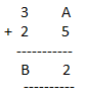Solution:

Say, A = 7 and we get,

7+5 = 12

In which one’s place is 2.

Therefore, A = 7

And putting 2 and carry over 1, we get

B = 6

Hence A = 7 and B = 6

2.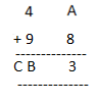Solution:

If A = 5 and we get,

8+5 = 13 in which ones place is 3.

Therefore, A = 5 and carry over 1 then

B = 4 and C = 1

Hence, A = 5, B = 4 and C = 1

3.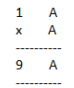Solution:

On putting A = 1, 2, 3, 4, 5, 6, 7 and so on and we get,

AxA = 6×6 = 36 in which ones place is 6.

Therefore, A = 6

4.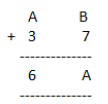Solution:

Here, we observe that B = 5 so that 7+5 =12

Putting 2 at ones place and carry over 1 and A = 2, we get

2+3+1 =6

Hence A = 2 and B =5

5.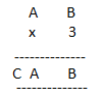Solution:

Here on putting B = 0, we get 0x3 = 0.

And A = 5, then 5×3 =15

A = 5 and C=1

Hence A = 5, B = 0 and C = 1

6.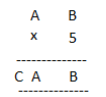Solution:

On putting B = 0, we get 0x5 = 0 and A = 5, then 5×5 =25

A = 5, C = 2

Hence A = 5, B = 0 and C =2

7.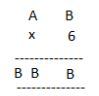Solution:

Here product of B and 6 must be same as ones place digit as B.

6×1 = 6, 6×2 = 12, 6×3 = 18, 6×4 =24

On putting B = 4, we get the ones digit 4 and remaining two B’s value should be44.

Therefore, for 6×7 = 42+2 =44

Hence A = 7 and B = 4

8.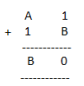Solution:

On putting B = 9, we get 9+1 = 10

Putting 0 at ones place and carry over 1, we get for A = 7

7+1+1 =9

Hence, A = 7 and B = 9

9.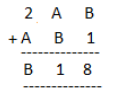Solution:

On putting B = 7, we get 7+1 =8

Now A = 4, then 4+7 =11

Putting 1 at tens place and carry over 1, we get

2+4+1 =7

Hence, A = 4 and B = 7

10.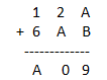Solution:

Putting A = 8 and B = 1, we get

8+1 =9

Now, again we add2 + 8 =10

Tens place digit is ‘0’ and carry over 1. Now 1+6+1 = 8 =A

Hence A = 8 and B =1

## Exercise 16.2 Page No: 260

1. If 21y5 is a multiple of 9, where y is a digit, what is the value of y?

Solution:

Suppose 21y5 is a multiple of 9.

Therefore, according to the divisibility rule of 9, the sum of all the digits should be a multiple of 9.

That is, 2+1+y+5 = 8+y

Therefore, 8+y is a factor of 9.

This is possible when 8+y is any one of these numbers 0, 9, 18, 27, and so on

However, since y is a single digit number, this sum can be 9 only.

Therefore, the value of y should be 1 only i.e. 8+y = 8+1 = 9.

2. If 31z5 is a multiple of 9, where z is a digit, what is the value of z? You will find that there are two answers for the last problem. Why is this so?

Solution:

Since, 31z5 is a multiple of 9.

Therefore according to the divisibility rule of 9, the sum of all the digits should be a multiple of 9.

3+1+z+5 = 9+z

Therefore, 9+z is a multiple of 9

This is only possible when 9+z is any one of these numbers: 0, 9, 18, 27, and so on.

This implies, 9+0 = 9 and 9+9 = 18

Hence 0 and 9 are two possible answers.

3. If 24x is a multiple of 3, where x is a digit, what is the value of x?

(Since 24x is a multiple of 3, its sum of digits 6+x is a multiple of 3; so 6+x is one of these numbers: 0, 3, 6, 9, 12, 15, 18, … . But since x is a digit, it can only be that 6+x = 6 or 9 or 12 or 15. Therefore, x = 0 or 3 or 6 or 9. Thus, x can have any of four different values.)

Solution:

Let’s say, 24x is a multiple of 3.

Then, according to the divisibility rule of 3, the sum of all the digits should be a multiple of 3.

2+4+x = 6+x

So, 6+x is a multiple of 3, and 6+x is one of these numbers: 0, 3, 6, 9, 12, 15, 18 and so on.

Since, x is a digit, the value of x will be either 0 or 3 or 6 or 9, and the sum of the digits can be 6 or 9 or 12 or 15 respectively.

Thus, x can have any of the four different values: 0 or 3 or 6 or 9.

4. If 31z5 is a multiple of 3, where z is a digit, what might be the values of z?

Solution:

Since 31z5 is a multiple of 3.

Therefore according to the divisibility rule of 3, the sum of all the digits should be a multiple of 3.

That is, 3+1+z+5 = 9+z

Therefore, 9+z is a multiple of 3.

This is possible when the value of 9+z is any of the values: 0, 3, 6, 9, 12, 15, and so on.

At z = 0, 9+z = 9+0 = 9

At z = 3, 9+z = 9+3 = 12

At z = 6, 9+z = 9+6 = 15

At z = 9, 9+z = 9+9 = 18

The value of 9+z can be 9 or 12 or 15 or 18.

Hence 0, 3, 6 or 9 are four possible answers for z.

Access Class-8 Chapter-wise Solutions: